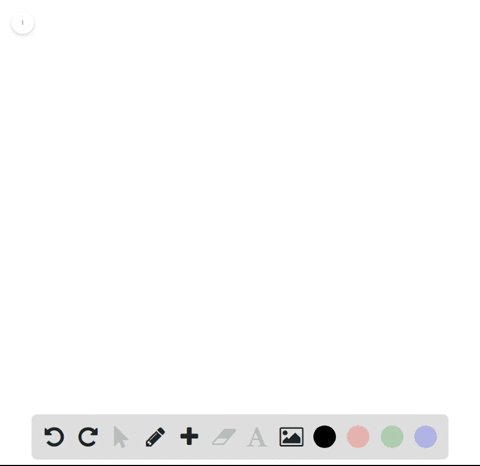### The speed of a runner increased steadily during t…

01:09
FL
Problem 12

(a) If $f(x) = \ln x$, $1 \le x \le 4$, use the commands discussed in Exercise 11 to find the left and right sums for $n$ = 10, 30, and 50.

(b) Illustrate by graphing the rectangles in part (a).

(c) Show that the exact area under $f$ lies between 2.50 and 2.59.

(A). $L 10 \approx 2.331630 R 10 \approx 2.747518 L 30 \approx 2.475238 R 30 \approx 2.613867 L 50 \approx$ 2.503364$R 50 \approx 2.586541$
(B). Click to see
(C). $4 \ln 4-3 \approx 2.54517744$

## Discussion

You must be signed in to discuss.

## Video Transcript

so improbable I explained how to compute or how to estimate the area under F by using eleven point and right in point. That's eleven Royce Somes for a general. Former, please take a look at that problems and you can plug in the specific A function here and the specific values here and for the answer of this problem.